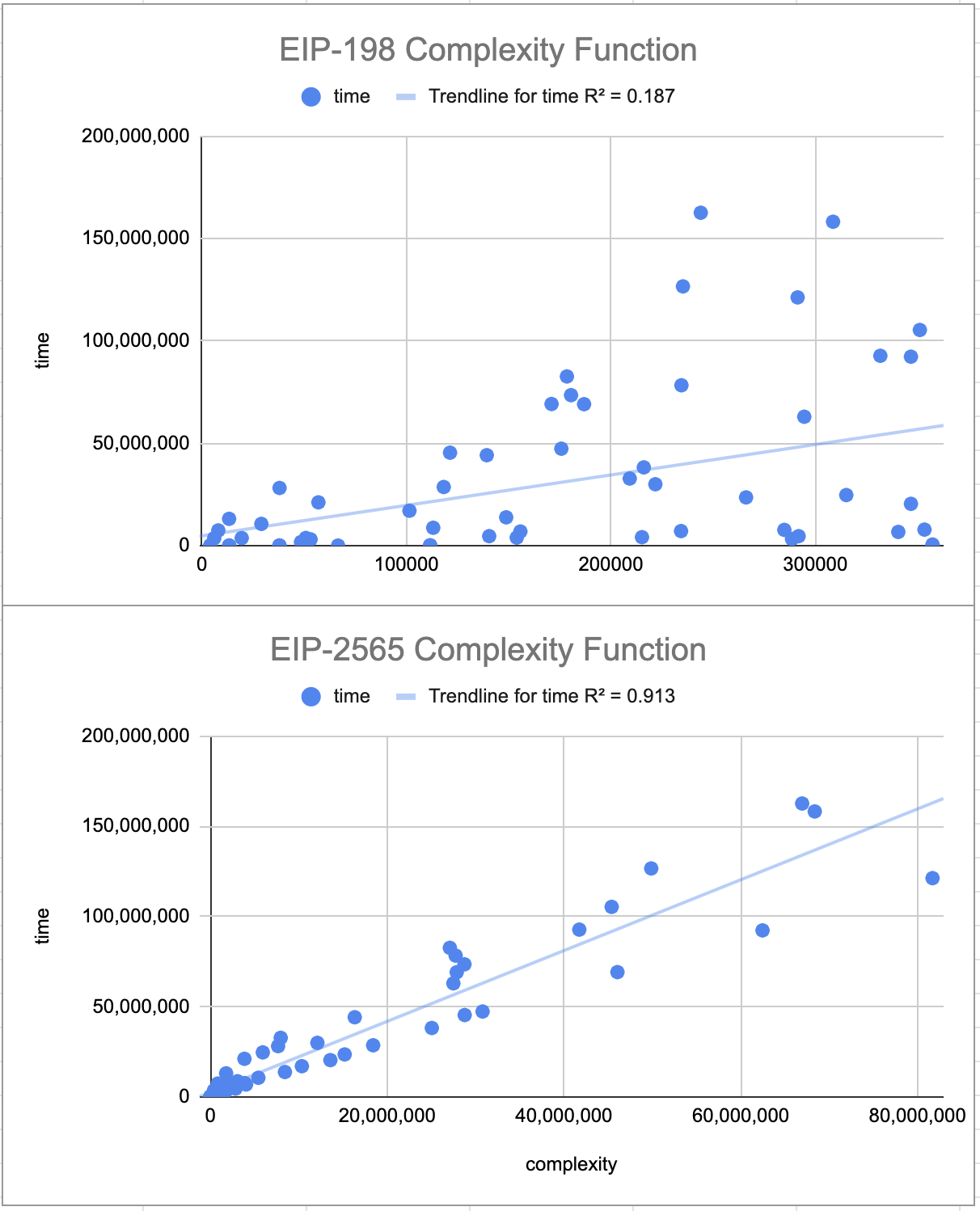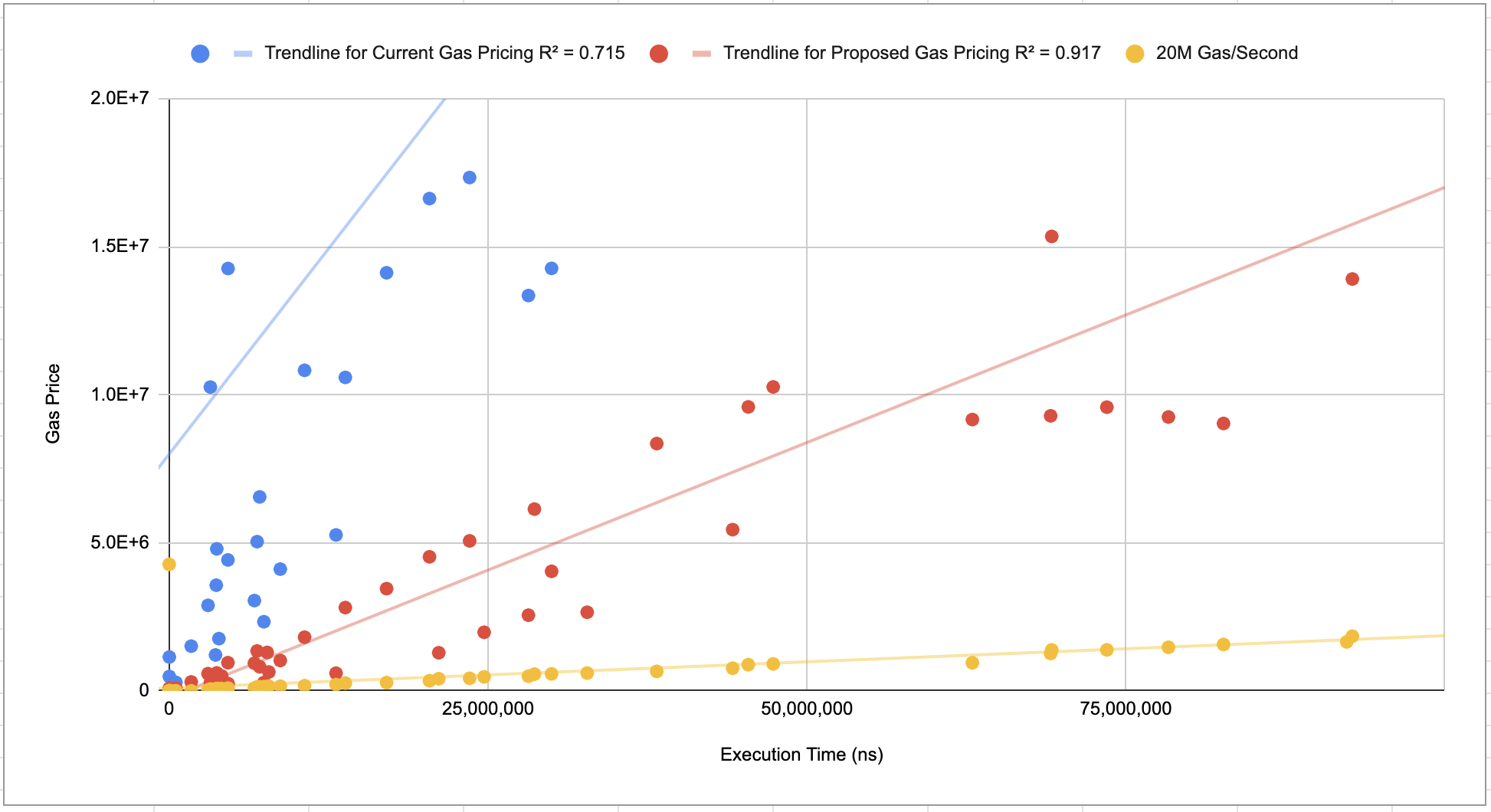# EIP-2565: ModExp Gas Cost

Authors Kelly Olson (@ineffectualproperty), Sean Gulley (@sean-sn), Simon Peffers (@simonatsn), Justin Drake (@justindrake), Dankrad Feist (@dankrad) 2020-03-20 EIP-198

## Simple Summary

Defines the gas cost of the ModExp (0x00..05) precompile.

## Abstract

To accurately reflect the real world operational cost of the ModExp precompile, this EIP specifies an algorithm for calculating the gas cost. This algorithm approximates the multiplication complexity cost and multiplies that by an approximation of the iterations required to execute the exponentiation.

## Motivation

Modular exponentiation is a foundational arithmetic operation for many cryptographic functions including signatures, VDFs, SNARKs, accumulators, and more. Unfortunately, the ModExp precompile is currently over-priced, making these operations inefficient and expensive. By reducing the cost of this precompile, these cryptographic functions become more practical, enabling improved security, stronger randomness (VDFs), and more.

## Specification

As of FORK_BLOCK_NUMBER, the gas cost of calling the precompile at address 0x0000000000000000000000000000000000000005 will be calculated as follows:

def calculate_multiplication_complexity(base_length, modulus_length):
max_length = max(base_length, modulus_length)
words = math.ceil(max_length / 8)
return words**2

def calculate_iteration_count(exponent_length, exponent):
iteration_count = 0
if exponent_length <= 32 and exponent == 0: iteration_count = 0
elif exponent_length <= 32: iteration_count = exponent.bit_length() - 1
elif exponent_length > 32: iteration_count = (8 * (exponent_length - 32)) + ((exponent & (2**256 - 1)).bit_length() - 1)
return max(iteration_count, 1)

def calculate_gas_cost(base_length, modulus_length, exponent_length, exponent):
multiplication_complexity = calculate_multiplication_complexity(base_length, modulus_length)
iteration_count = calculate_iteration_count(exponent_length, exponent)
return max(200, math.floor(multiplication_complexity * iteration_count / 3))


## Rationale

After benchmarking the ModExp precompile, we discovered that it is ‘overpriced’ relative to other precompiles. We also discovered that the current gas pricing formula could be improved to better estimate the computational complexity of various ModExp input variables. The following changes improve the accuracy of the ModExp pricing:

### 1. Modify ‘computational complexity’ formula to better reflect the computational complexity

The complexity function defined in EIP-198 is as follow:

def mult_complexity(x):
if x <= 64: return x ** 2
elif x <= 1024: return x ** 2 // 4 + 96 * x - 3072
else: return x ** 2 // 16 + 480 * x - 199680


where is x is max(length_of_MODULUS, length_of_BASE)

The complexity formula in EIP-198 was meant to approximate the difficulty of Karatsuba multiplication. However, we found a better approximation for modelling modular exponentiation. In the complexity formula defined in this EIP, x is divided by 8 to account for the number of limbs in multiprecision arithmetic. A comparison of the current ‘complexity’ function and the proposed function against the execution time can be seen below:The complexity function defined here has a better fit vs. the execution time when compared to the EIP-198 complexity function. This better fit is because this complexity formula accounts for the use of binary exponentiation algorithms that are used by ‘bigint’ libraries for large exponents. You may also notice the regression line of the proposed complexity function bisects the test vector data points. This is because the run time varies depending on if the modulus is even or odd.

### 2. Change the value of GQUADDIVISOR

After changing the ‘computational complexity’ formula in EIP-198 to the one defined here it is necessary to change QGUADDIVSOR to bring the gas costs inline with their runtime. By setting the QGUADDIVISOR to 3 the cost of the ModExp precompile will have a higher cost (gas/second) than other precompiles such as ECRecover.### 3. Set a minimum gas cost to prevent abuse

This prevents the precompile from underpricing small input values.

## Test Cases

There are no changes to the underlying interface or arithmetic algorithms, so the existing test vectors can be reused. Below is a table with the updated test vectors:

Test Case EIP-198 Pricing EIP-2565 Pricing
modexp_nagydani_1_square 204 200
modexp_nagydani_1_qube 204 200
modexp_nagydani_1_pow0x10001 3276 341
modexp_nagydani_2_square 665 200
modexp_nagydani_2_qube 665 200
modexp_nagydani_2_pow0x10001 10649 1365
modexp_nagydani_3_square 1894 341
modexp_nagydani_3_qube 1894 341
modexp_nagydani_3_pow0x10001 30310 5461
modexp_nagydani_4_square 5580 1365
modexp_nagydani_4_qube 5580 1365
modexp_nagydani_4_pow0x10001 89292 21845
modexp_nagydani_5_square 17868 5461
modexp_nagydani_5_qube 17868 5461
modexp_nagydani_5_pow0x10001 285900 87381

Geth

Python

## Security Considerations

The biggest security consideration for this EIP is creating a potential DoS vector by making ModExp operations too inexpensive relative to their computation time.

EIP-198

Copyright and related rights waived via CC0.